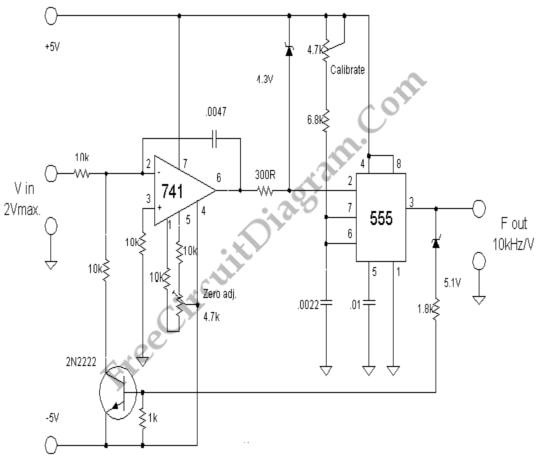# 555 – 741 IC Voltage To Frequency ConverterThis voltage to frequency converter (VFC) circuit uses 555 IC and 741 op-amp as the main components. Up to 20kHz oscillation can be produced by this circuit. Here is the schematic diagram:The zero adjustment is used to adjust the lowest frequency, short the input to ground and adjust this pot to obtain the lowest frequency limit. This circuit uses symmetric supply +5, 0 (ground), and -5V. The output is a squarewave with the frequency varies in accordance with the input voltage at 1okHz per Volt conversion. [Circuit’s schematic diagram source: lh-electric.4t.com]